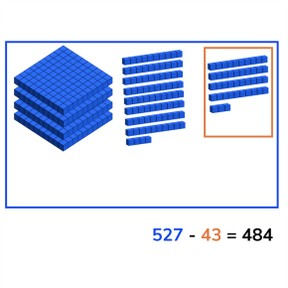Subtraction to 1,000 crossing hundreds

# Subtraction to 1,000 crossing hundreds

Subtract numbers to 1,000 crossing hundreds.8,000 schools use Gynzy92,000 teachers use Gynzy1,600,000 students use Gynzy

## General

Students learn to subtract numbers to 1,000 crossing hundreds.

## Standards

CCSS.Math.Content.2.NBT.B.7

## Learning objective

Students will be able to subtract numbers to 1,000 crossing hundreds.

## Introduction

Using the interactive whiteboard, show the math problem and the three students who each have an answer. Ask the class to determine which student has the correct answer. Ask the class to explain their strategy. Repeat this.

## Instruction

Explain that you can subtract by counting. You first count how many there are in total, this is the first number of the subtraction problem (the minuend). Then you count how many are taken away, which is the second number of the subtraction problem (the subtrahend). When you take the second from the first you are left with the difference. Show this on the interactive whiteboard using the blocks, and discuss the different problems. Check that students are able to solve a few problems on their own or in pairs with the given problems with blocks.Ask them to say the problem they are solving out loud, and to say the steps they are taking out loud. Next explain that you can solve these subtraction problems in different ways. Show that you can use a number line to subtract. You can also split the subtrahend and take away the hundreds, tens, and ones in steps. You can also choose to take away the subtrahend in one step. Emphasize to students that they should first carefully look at the problem and then decide which method to use, because all of them work, but some are easier to use on different math problems. Ask students to solve a few problems individually or in pairs, explaining their strategy. Finally walk through the steps of a story problem with the class. Explain that you first determine what kind of math problem it is, then you determine which numbers are important for the problem. You say or write the problem and finally solve the problem. Do one story problem as a class and then ask students to solve another individually or in pairs.

Check that students are able to subtract numbers to 1,000 crossing hundreds by asking the following questions:
- What steps do you take to solve a story problem?
- How do you solve 628 - 73 and 715 - 361?

## Quiz

Students are given problems in which they must solve using visual support, solve an abstract problem, and story problems. Encourage students to explain their strategy and walk through the steps of the subtraction problems.

## Closing

Discuss with students that it is important to be able to subtract numbers to 1,000 so that you can solve math problems and determine how much you have left. Show the counter on the interactive whiteboard that shows a product, and how much it costs. Then ask students to look at the piggy bank and determine how much money they have left after purchasing the item.

## Teaching tips

Students who have difficulty with subtraction can make use of the number line to support their subtraction. Remind students that they can take different sized steps on the number line. They can also use a piece of scratch paper to solve the problem in multiple steps.

## Instruction materials

MAB blocks or manipulatives, number line.

### The online teaching platform for interactive whiteboards and displays in schools

• Save time building lessons

• Manage the classroom more efficiently

• Increase student engagement